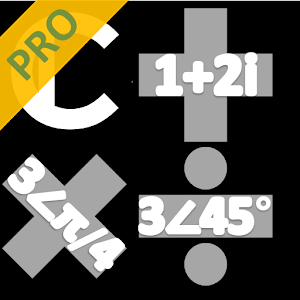# Complex Number Calculator PRORating: 0/5

The most accurate and the most comprehensive Complex Number Calculator (and with special features useful to electrical engineering) to compute scientific/mathematical expressions containing real, imaginary and, in general, complex numbers in standard (rectangular) a+bi and polar (phasor) r∠(θ) forms such as

(1/2 + 2i )(3 + 4i) + 3∠(π/4) + sin(i)cos(2 + 3i)^(4 – 2i) / ln(2+i)

The calculations can be performed in RAD (radians) or DEG (degrees) mode.

With the best user interface, this comprehensive complex number calculator optionally converts the result or any complex number in standard form to polar and other modular forms and vice versa (long press i or ∠ to see all forms of the calculated result).

The result can be displayed with fixed, scientific and engineering notations.

This exceptionally powerful complex/scientific calculator supports all main mathematical operations (addition, subtraction, multiplication and division) and functions (power, exponent, logarithm, trigonometric, hyperbolic and their inverses, etc.) with real, imaginary or complex numbers as their arguments.

Additionally, this scientific complex number calculator supports the Gamma function, Γ, and the psi function, Ψ, and the zeta function, ζ, with real/imaginary/complex numbers as their arguments.

You can also calculate some quantities used in electrical engineering involving complex numbers, i.e., impedance, by selecting expressions and long pressing Σ.

——————
Important!
As the value of trigonometric functions depend on the angle mode chosen, by selecting RAD or DEG the complex number calculator, needless to say, displays the computed values correspondingly. Note that, because many functions of complex numbers are evaluated using the trig functions via Euler’s identity, e^(ix) = cos(x)+ i*sin(x), their values depend on the angle mode selected although they may look non-trigonometric. For example, the value of e^2 is the same regardless of the angle mode selected. However the value of e^i depends on the angle mode because it has the same value as cos(1)+ i*sin(1).
Other complex numbers calculators may not give you the correct results when calculating with complex numbers in DEG mode.
——————
This scientific calculator also lets you do statistics with one or two dimensional real data and do a lot more.

Description of additional scientific features and detailed built-in instruction is provided in the menu accompanying this scientific calculator/complex number calculator.
Download it here